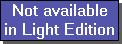The SDL Component Suite is an industry leading collection of components supporting scientific and engineering computing. Please visit the SDL Web site for more information....## FindCentersUnit: SDL_math2 Class: none Declaration: procedure FindCenters (InMat: TMatrix; RowLo, RowHi: integer; NumCent: integer; var Centers: TMatrix; var MeanDist: double);

If a matrix is seen as a collection of measurements (rows=objects, columns=variables) it is sometimes useful to calculate prototype objects which are representative for the whole data set. This calculation of the prototypes is equal to the searching of clusters in an n-dimensional space, where n is the number of variables per object.

The procedure FindCenters calculates the specified number of centers NumCent by performing an agglomerative clustering (average linkage) which is aborted when NumCent clusters have been built. The parameters RowLo and RowHi determine which objects are used for the calculation.

The centers are calculated as follows: First, the two objects are searched which exhibit the smallest distance of each other. This pair of data is now replaced by their center of gravity. The calculation of the center of gravity allows for the number of data points already used in preceding unifications. Now the unification is repeated until only NumCent points are left. These points are equal to the inquired centers.

The procedure FindCenters returns the found prototypes in the matrix Centers and the mean distance between them in the parameter MeanDist. The user has to take care of by himself that the matrix Centers is large enough to accept all calculated prototype points (i.e. Centers has to have at least NumCent rows and InMat.NrOfCOlumns columns). If the matrix Centers is too small the result will be truncated accordingly. If it is too large, rows from NumCent +1 on are not defined and excess columns are set to zero values.

FindCenters increments the global variable ProcStat and calls the feedback routine MathFeedBackProc in order to allow feedback to the user during time consuming calculations.

 Hint 1: The time it takes to compute the prototypes increases with the third power of the number of objects. Thus it is strongly recommended not to use this algorithm for more than approx. 200 objects.

 Hint 2: FindCenters stores the original data of the matrix in a file named MAT.\$\$\$ in order to reload the data after the calculation of the prototype points. The auxiliary file MAT.\$\$\$ is automatically deleted after the execution of FindCenters.

 Example: FINDCENT.DPR The example application FINDCENT shows the usage of FindCenters to find centers in a two-dimensional data array. Note, that FINDCENT.DPR uses the components RCHART and NUMLAB for the user interface, which are not included in the MATH2 package (you can download it from the SDL Web site: http://www.lohninger.com/download_sdlsuite.html).

 Example: This method is used in the following example program (see http://www.lohninger.com/examples.html for downloading the code): findcent

Last Update: 2015-Mar-26